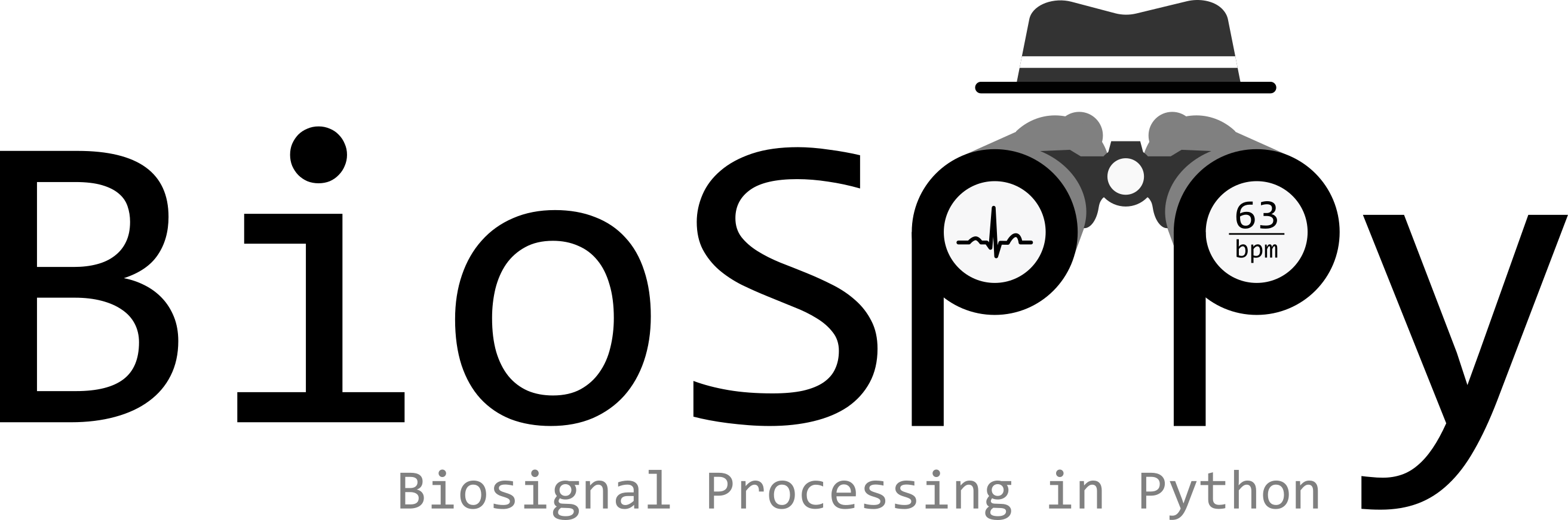# Welcome to `BioSPPy`¶`BioSPPy` is a toolbox for biosignal processing written in Python. The toolbox bundles together various signal processing and pattern recognition methods geared torwards the analysis of biosignals.

Highlights:

• Support for various biosignals: BVP, ECG, EDA, EEG, EMG, Respiration
• Signal analysis primitives: filtering, frequency analysis
• Clustering
• Biometrics

Contents:

## Installation¶

Installation can be easily done with `pip`:

```\$ pip install biosppy
```

## Simple Example¶

The code below loads an ECG signal from the `examples` folder, filters it, performs R-peak detection, and computes the instantaneous heart rate.

```import numpy as np
from biosppy.signals import ecg

# load raw ECG signal
signal = np.loadtxt('./examples/ecg.txt')

# process it and plot
out = ecg.ecg(signal=signal, sampling_rate=1000., show=True)
```